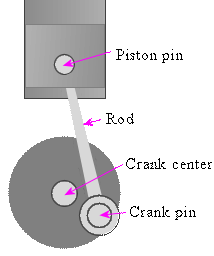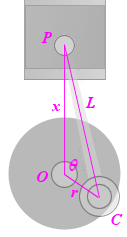Search IntMath
Close

450+ Math Lessons written by Math Professors and Teachers

5 Million+ Students Helped Each Year

1200+ Articles Written by Math Educators and Enthusiasts

Simplifying and Teaching Math for Over 23 Years

#### Tips, tricks, lessons, and tutoring to help reduce test anxiety and move to the top of the class.

Home | Math Display Experiments | Curve Shape for the Movement of a Piston

Page by Murray Bourne, IntMath.com. Last updated: 07 Jul 2019

# Curve Shape for the Movement of a Piston

When a piston moves through a cylinder the resulting motion is almost sinusoidal if the crank has a small radius compared to the length of the rod.

However, if the crank radius is large compared to the rod length, the curve shape is not at all sinusoidal.

This applet allows you to explore this concept.

## Piston partsWe represent the distances and points involved as follows:L is the (fixed) length of the rod

x is the distance from the center of the crank (O) and the piston pin (P).

r is the distance OC.

theta is the angle /_POC.

Now, using the Cosine Rule from basic trigonometry, we have:

L^2 = r^2 + x^2 - 2rx cos(theta)

Solving this for x, and using the fact cos^2 theta = 1 - sin^2 theta, we get the following, which is the equation for the movement of a piston driven by a circular crank:

x=rcosA+sqrt(L^2-r^2sin^2 theta)

We can see from this last equation that if L is large compared to r, the expression under the square root becomes close to sqrt(L^2) = L (a constant), and the motion will be close to x=rcosA+L, which is sinusoidal.

## The piston applet

In this applet, the lighter colored curve is a "pure" sine curve. You can see how the darker curve is not quite the same.

Change the sliders to vary the crank radius and the rod length.

See what happens when amplitude = rod length.